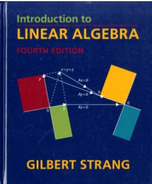Introduction To Linear Algebra - 4 Edition - Chapter 3.6 - Problem 14
Register Now

Join StudySoup

Get Full Access to Introduction To Linear Algebra - 4 Edition - Chapter 3.6 - Problem 149780980232714

# Without computing A, find bases for its four fundamental subspaces: [ L 0 0] [1 2 3 4]

Introduction to Linear Algebra | 4th Edition

Problem 14

Without computing A, find bases for its four fundamental subspaces: [ L 0 0] [1 2 3 4] A= 6 1 0 0 1 2 3 . 9810012

Accepted Solution
Step-by-Step Solution:
Step 1 of 3

3.2 The Mean Value Theorem &Rolle's Theorem The MeanValue Theorem Letf be a function that satisfies the following: 1. f is continuous on the interval [a, b] 2. f is differentiable on the i(a, b)l Then there is a numbcin a,b)such that: f'(c) = f(bf(a) b-a Rolle's Theorem Letf be a function that satisfies the following: n. f is continuous on the interval [a, b] l.2.f is differentiable on the (a,b)val "f3. f(a)= f(b) Then there is a numbcin a,b)such that: 1rec)= o Problem6 Find all numbec,that satisfy the conclusion ofthe MeanValueTheorem for the 1 function/(x)=__i_ontheinterval[2,5]. [~, b] f{K): '-f(t-)

###### Chapter 3.6, Problem 14 is Solved

Step 2 of 3

Step 3 of 3

Unlock Textbook Solution